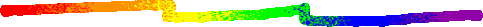Have fun performing these mathemagic tricks
for your family and friends!MOBIUS MYSTERY

This mathemagic trick is named after the 19th-century German mathematician and astonomer, August Ferdinand Mobius. Over 150 years ago, Mobius traveled around Germany performing mathemagic shows and this trick was one of his favorites.

What You Need
A pair of scissors
Cellophane tape
3 Strips of paper (Cut each strip about 3 inches by 28 inches)
- Tape the first strip together with no twist
- Tape the second strip together with a half twist
- Tape the third strip together with a half twist

What To Do
Carefully cut the first two strips straight down the middle until you get back to where you started. If you cut correctly, you will get 2 separate circles and one big circle. Carefully cut the third strip one-third of the way from the right edge. Keep cutting until you get back to where you started (it will take twice as long), and you will get two circles connected like a chain.

Other Things To Do
Try cutting down the center of the big circle and you will be surprised with the result!

The Mathemagical Secret
This trick uses a kind of mathematics called topology. Topology is the study of shapes and what happens to those shapes when they are changed by folding, pulling, bending, twisting, or stretching.

From the book MATHEMAGIC by Raymond Blum
Sterling Publishing Company, Inc., New York, NY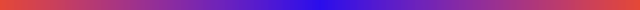CURLEY'S TRICK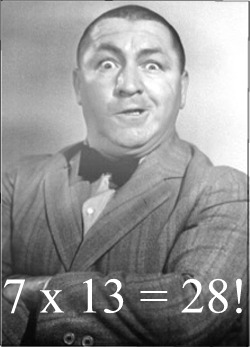Your friend's are thoroughly confused when you "prove" that 7 X 13 = 28!

What You Need
Paper and pencil

What To Do
Only a number magician could prove that 7 X 13 = 28. Here are three different ways to prove it. If you talk fast enough, you will be able to fool your family and friends!

FIRST WAY -- MULTIPLICATION

13                      7 times 3 equals 21 and
X 7                           7 times 1 equals 7.
21                               21 + 7 = 28
+ 7
28                             So: 7 X 13 = 28!!

SECOND WAY -- DIVISION

13            7 does not divide into 2, but it does
7)28                divide into 8 one time. Put down
7             the 1 and subtract 7. That leaves 21.
21                    7 divides into 21 three times.
21
You multiply to check division.

13
So,if   7)28   then    7 X 13 = 28!!

THIRD WAY -- ADDITION

Multiplication is repeated addition so 7 X 13
is seven thirteens added together.

(22)   13    (21)
(23)   13    (18)     Add up the column of 3's and get 21.
(24)   13    (15)           Then add down the column of 1's
(25)   13    (12)             and get 7 more (21 + 7 = 28).
(26)   13    ( 9 )
(27)   13    ( 6 )                         So: 7 X 13 = 28!!
(28) +13    ( 3 )
28

From the book MATHEMAGIC by Raymond Blum
Sterling Publishing Company, Inc., New York, NYCALCULATOR MAGIC

Carefully follow the directions, and your telephone number will magically appear in your calculator's display!

What You Need
A calculator

What To Do
1. Enter into your calculator the first 3 digits of your phone number (not area code).
2. Multiply by 80  (Always push = after you +, -, x or �)
4. Multiply the result by 250
5. Add the last four digits of your number to the result
6. Add the last four digits again
7. Subtract 250
8. Divide by 2

Does your answer look familiar? If you didn't get your phone number, try again.

Variations
Try other phone numbers. It will work every time!

The Mathemagical Secret:
This trick was written using a kind of mathematics called algebra. Multiplying by 80 and 250 and then dividing by 2 is the same as multiplying by 10,000. This moves the first 3 digits of your phone number over and to the left of the ten-thousands place. Adding the last 4 digits of your phone number twice and then dividing by 2 is the same as adding the last four digits once. Every other operation is mathematical hocus pocus and adds an extra 250 to the total. Subtracting 250 reveals your phone number at the very end.

Similar tricks appear in the book MATHAMANIA by Raymond Blum
Sterling Publishing Company, Inc., New York, NYNUMBER SPIRITS

Three cards are removed from the deck at random and they are all Aces!  Then the "Number Spirits" are summoned and the fourth Ace mysteriously appears!

What You Need
A deck of playing cards

Preparation
Put an 8 card in the eighth position down from the top of the deck and put the four Aces in the ninth, tenth, eleventh, and twelve positions.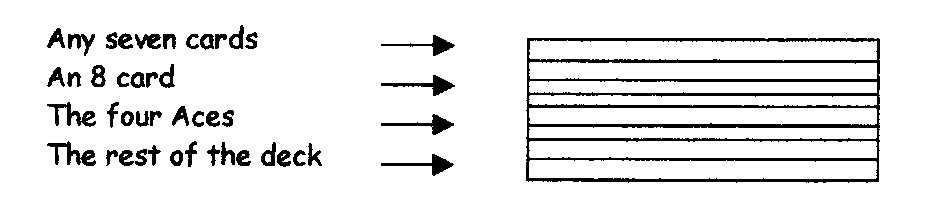What To Do
1.  Ask your friend for a number between  10 and 20.  (10 and 20
are not between.)   Example:17
2.  Deal that number of cards into a small pile, one card at a time.
Place the rest of the deck next to the small pile.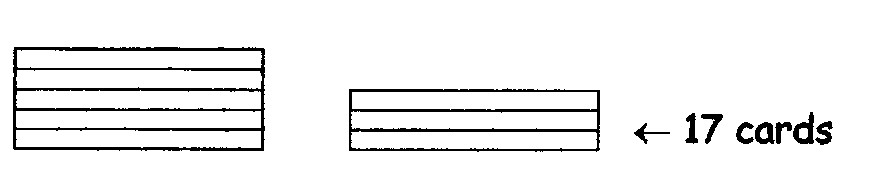3.  Ask your friend to find the sum of the digits of his number.
17 ---> 1 + 7 = 8

4.  Return that many cards to the top of the big pile, one card
at a time.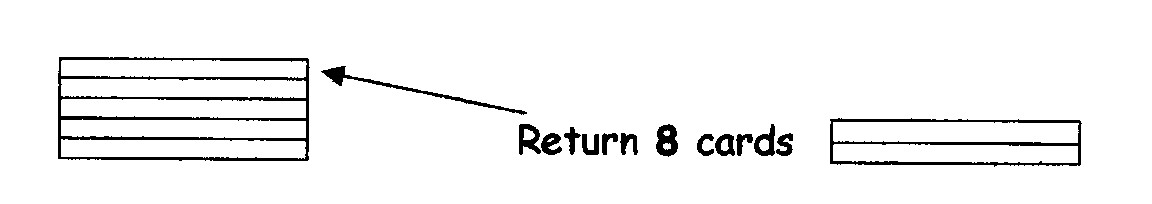5.  Put the small pile on top of the big pile.  The top card will be the first Ace!
Turn this card face up, show your friend, and set it aside.
6.  Repeat steps 1 - 5 with two different numbers between 10 and 20 to remove
two more Aces.

Finally, pretend to do some supernatural hocus pocus as you ask the "Number Spirits" for a sign to help you find the last Ace.  Pretend they tell you to turn over the top card.  It will be an 8 card.  Count down eight more cards and the eighth card will be the fourth Ace!

The Mathemagical Secret
Any number between 10 and 20 minus the sum of its digits always equals 9.

From the book MATHEMAGIC by Raymond Blum
Sterling Publishing Company, Inc., New York, NYPSYCHIC PREDICTION
(The 3 1/2 OF CLUBS using the Ace of Hearts)

You can amaze your friends by looking into the future and predicting which card will be chosen from the deck!

What You Need
A deck of playing cards, a calculator, paper and pencil

Preparation
Put the Ace of Hearts in the eighth position down from the TOP of the deck.

What To Do
Tell your friend you are going to predict which card he will choose from the deck of cards. Secretly write ACE OF HEARTS! on a piece of paper, fold it several times, and set it aside until later. Shuffle the cards. (See Below)

Tell your friend to think of a number that is very easy to remember and has 1, 2, 3, or 4 digits.
Examples: His age, lucky number, address, favorite number, etc.

Clear the calculator, hand it to your friend, and have him:
1. Enter his number in the calculator.
2. Multiply that number by 3 and then push the equal sign.
3. Add 24 to that result and then push the equal sign.
4. Multiply that answer by 2 and then push the equal sign.
5. Divide that result by 6 and then push the equal sign.
6. Subtract his original number and then push the equal sign.

Remind your friend that he was free to choose any number from 0-9,999 when he started. Then, after some adding, subtracting, multiplying, and dividing he ended up with another random number. (It will always be 8.) Tell your friend to count that many cards down from the top of the deck, and turn over the LAST CARD. (The Ace of Hearts) Open your prediction and it will match his chosen card!!!

The Mathemagical Secret
This trick was written using a kind of mathematics called algebra. Multiplying by 3 and then by 2 is the same as multiplying by 6. Dividing by 6 cancels those operations. Every other operation is mathematical hocus-pocus that eliminates your friend's original number and guarantees the final total will always be 8.

Shuffling The Deck
There is a way of shuffling the cards so the Ace of Hearts remains in the eighth position down from the top of the deck. Divide the deck into two piles and riffle the ends together allowing the two piles to interweave. Let the top ten to twelve cards fall last and the Ace of Hearts will remain in the eighth position no matter how many times you shuffle. Practice this many times before trying it with a friend.

From the book MATHEMAGIC by Raymond Blum
Sterling Publishing Company, Inc., New York, NYMAGIC SPELL

Your friend secretly chooses a card from a deck of cards.  When a magical phrase is spelled out, your friend's secret card mysteriously appears!

What You Need
A deck of playing cards

What To Do
1. Cut the deck into seven piles and lay them face down on the table.
2. Ask your friend to point to one of the piles. (It doesn't matter which one she chooses.) Gather together all the piles she did not choose into one pile, and then put her chosen pile on the TOP.
3. Tell her to secretly look at the top card, memorize it, and return it to the TOP of the deck.
4. Ask her for any number between 20 and 30.  (20 and 30 are not between.)

Example: 23

5. Deal that number of cards into a small pile, one card at a time.  Place the rest of the deck next to the small pile.6. Ask your friend to find the sum of the digits of her number.
23 --->  2 + 3 = 5
7. Return that many cards from the small pile to the top of the big pile, one card at a time.8. Put the small pile on top of the big pile.

Finally, hand your friend the deck.  As you slowly spell the magical phrase "h-o-c-u-s  p-o-c-u-s  a-l-a-c-a-z-a-m", tell her to turn over one card for each letter.  When she turns over the LAST CARD, it will be her secret card!

The Mathemagical Secret
Any number between 20 and 30 minus the sum of its digits always equals 18.
"Hocus pocus alacazam" has exactly 18 letters.

From the book MATHEMANIA by Raymond Blum
Sterling Publishing Company, Inc., New York, NYCALCULATOR RIDDLES

Your calculator is a remarkable little machine. You've always known that it can perform mathematical calculations faster and with more accuracy than most humans, but did you know that it can also talk?

Yes, it's true! Your calculator will talk to you if you push the right buttons. For example, your calculator will tell you its name if you push clear and then carefully push 353 x 9 + 5,000 - 459 = Just turn your calculator upside down, and it will tell you!

Now that you and BILL have been properly introduced, it's time to have some fun! Use the calculator alphabet to help you find the answers to the math jokes and math riddles. If you don't understand an answer, look at the explanation in the answer section or just ask your calculator!

THE CALCULATOR ALPHABET

0 - O,   1 - I,   2 - Z,   3 - E,   4 - h   5 - S,   6 - g,   7 - L,   8 - B,   9 - G

What You Need
A calculator

1. What is the only thing that gets larger the more you take away?
25,000 - 68 - 952 - 8,956 - 11,320 =
2. A pet store owner has 19 eels. All but 9 were sold. How many eels does the pet store owner have left?
337.8 x 17 - 9 =
3. Who weighs more, Lee the 5-foot butcher or Bob the 7-foot wrestler?
5 x 7 x 10 - 13 =
4. A barrel of water weighed 75 pounds, but after somebody put something in it, it weighed only 20 pounds.
What was put in the barrel?
500 x 100 + 4,000 - 300 + 4 =
5. What is the largest number that will fit in your calculator's display?
99,999,999 / 9 - 11,058,162 + 656,060 =
6. What did seven do that made all the other numbers afraid of it?
7 x .07 / .7 x 7 + 1.9 =
7. Take two eggs from three eggs and what do you have?
9,992 x .2 x 3 - 2 =
8. How many seconds are in a year?
31,557,600 / 1,000,000 - 26.3476 =
9. How much dirt is in a hole that is 5-feet deep, 2-feet wide and 3-feet long?
5 x 2 x 3 - 30 =
10. Which would you rather have, and old one-hundred-dollar bill or a brand-new one?
100 x 77 + 118.001 - 100 =

From the book MATHAMZING by Raymond Blum
Sterling Publishing Company, Inc., New York, NYThis number trick is easy to learn and fun to perform for others. You will amaze your family and friends with your supernatural powers when you mysteriously reveal the number that has been secretly chosen!

What You Need
5 index cards

Preparation
1. Copy the following numbers on five index cards. Write the numbers exactly as they are shown.

CARD 1
1     3     5     7
9   11   13   15
17   19   21   23
25   27   29   31

CARD 2
2     3     6     7
10   11   14   15
18   19   22   23
26   27   30   31

CARD 3
4     5     6     7
12   13   14   15
20   21   22   23
28   29   30   31

CARD 4
8     9   10   11
12   13   14   15
24   25   26   27
28   29   30   31

CARD 5
16   17   18   19
20   21   22   23
24   25   26   27
28   29   30   31

2. Read the directions below and then practice the trick by yourself. When you have successfully worked the trick two or three times, you are ready to perform it for others.

What To Do
1. Ask your friend to secretly think of a number from 1 to 31.
2. Give him the 5 index cards and ask him to hand you each card that has his secret number on it.
3. As your friend hands you each card, glance at the number in the top left-hand corner.
4. Mentally add these numbers. Their sum will be his secret number.
5. Finally, hold these cards to your forehead, close your eyes, and pretend the cards are speaking to you as you reveal your friend’s secret number!

Example
Suppose your friend chooses 19 as the secret number. He would hand you each card that has a 19 on it (Card 1, Card 2, & Card 5). The sum of the numbers in the top left-hand corner of each card ( 1 + 2 + 16 ) is 19.

The Mathemagical Secret
This trick uses the binary number system which is based on the number 2. Look at the number in the top left-hand corner of each card: 1, 2, 4, 8, & 16. Each number has been multiplied by 2 to get the next number. They are called powers of 2. The rest of the numbers on each card have these powers of 2 as parts of their numbers. For example, look at the card with the 8 in the top left-hand corner. The rest of the numbers on that card have 8 as part of their numbers. 9 = 8 + 1, 10 = 8 + 2, 11 = 8+ 2 + 1, 12 = 8 + 4, etc.

Other Things To Do
Because the cards are numbered up to 31, you can have someone choose a secret number that is an important date like an anniversary or a birthday.

From the book MATHAMUSEMENTS by Raymond Blum
Sterling Publishing Company, Inc., New York, NYPAIR-A-DICE

Your friend rolls two dice when you are not looking. After she works a few problems on a calculator, you are able to reveal the two top numbers on the dice!

What You Need
2 dice, A calculator, Paper and pencil

What To Do
When your back is turned, have a friend:

1. Roll two dice.
2. Multiply the top number on the first die by 5, using a calculator or paper and pencil.
4. Double that total.
5. Add that result to the top number on the second die.

Finally, ask your friend for her final total. Just subtract 39 from that total and the top numbers on the dice will magically appear!

The Mathemagical Secret:
Multiplying by 5 and then doubling is the same as multiplying by 10. This puts the number on the first die in the tens place. Adding the number on the second die puts that number in the ones place. Every other operation is mathematical hocus pocus and adds an extra 39 to the total. Subtracting 39 reveals the two top numbers on the dice.

From the book MATHAMAZING by Raymond Blum
Sterling Publishing Company, Inc., New York, NYCALCULATOR MAGIC Example

Jennifer's phone number is 836-7521.

1. Her phone number's first three digits are 836
2. 836 x 80 = 66,880
3. 66,880 + 1 = 66,881
4. 66,881 x 250 = 16,720,250
5. 16,720,250 + 7,521 = 16,727,771
6. 16,727,771 + 7,521 = 16,735,292
7. 16,735,292 - 250 = 16,735,042
8. 16,735,042 2 = 836-7521 -- Jennifer's phone number!

PSYCHIC PREDICTION Example

1. Your friend enters the number 100
2. 100 x 3 = 300
3. 300 +24 = 324
4. 324 x 2 = 648
5. 648 /6 = 108
6. 108 - 100 = 8

THE FINAL ANSWER WILL ALWAYS BE 8!

1. (hOLE) hole -- When you take away more dirt, the hole gets larger!
2. (9EELS) 9 eels -- 9 eels were not sold so there are 9 eels left!
3. (LEE) Lee -- Lee the butcher weighs meat all day long so he weighs more!
4. (hOLES) Holes -- 55 pounds of water leaked out!
5. (GOOGOL) googol -- A googol is a 1 followed by 100 zeroes so it has 101 digits!
6. (8,9) -- 7 (seven) 8 (ate) 9 (nine)!
7. (2 EGGS) 2 eggs. You took 2 eggs so you have 2 eggs!
8. (12S) 12 seconds -- January 2, February 2, March 2, etc.!
9. (0) 0 -- No matter how you turn the calculator, there is no dirt in a hole!
10. (100-BILL) \$100 bill. It is worth \$99 more than the new one(\$1)!

PAIR-A-DICE Example
PAIR-A-DICE Example

1. Your friend rolls a 2 and a 6.
2. 2 x 5 = 10.
3. 10 + 12 = 22
4. 22 x 2= 44
5. 44 + 6 = 50
6. 50 + 15 = 65

65 - 39 = 2 6. The number on the top of the first die is 2, and the number on the top of the second die is 6!